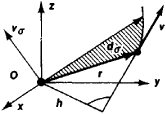# areal velocity

Also found in: Wikipedia.

## areal velocity

The velocity at which the radius vector sweeps out unit area. It is constant for motion in an elliptical orbit. See Kepler's laws.
Collins Dictionary of Astronomy © Market House Books Ltd, 2006
The following article is from The Great Soviet Encyclopedia (1979). It might be outdated or ideologically biased.

## Areal Velocity

a quantity characterizing the rate of increase of the area swept out by the radius vector r of a moving point. The radius vector is a vector drawn to the point from some fixed center O. If the area increases by during the infinitesimal length of time dt (see Figure 1), then the numerical value of the areal velocity is vσ = dσ/dt.Figure 1

The relation between the areal velocity and the speed v of the point is given by the equation vσ = vh/2, where h is the length of the perpendicular dropped from O to the direction of the vector v. In other words, the areal velocity is equal to half the moment of the velocity about O. The areal velocity can be represented as a vector vσ = (r × v)/2. In the case of the motion of a point along a plane curve, vs = (1/2)r2dΦ/dt, where r and Φ are the polar coordinates of the point. The derivative of the areal velocity with respect to time is sometimes called the areal acceleration wσ of the point; wσ = (r × w)/2, where w is the acceleration of the point.

The concept of areal velocity plays an important role in the study of motion under the influence of a central force—that is, a force whose line of action always passes through a center O. An example is the force of gravitational attraction; in this case, the areal velocity is constant. In particular, the areal velocity is constant in the case of the motion of planets (Kepler’s second law), artificial earth satellites (if the gravitational force is regarded as directed toward the earth’s center), and space vehicles.

S. M. TARG